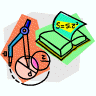## Mathematics, Department of## Faculty Publications, Department of Mathematics

8-11-2022

#### Citation

http://arxiv.org/abs/2208.05653v1

CC-BY-NC-ND

#### Abstract

We study the Hodge-Riemann property (HRP) for graded Artinian Gorenstein (AG) algebras. We classify AG algebras in codimension two that have HRP in terms of higher Hessian matrices and positivity of Schur functions associated to certain rectangular partitions.

In this paper we introduce the Hodge Riemann property (HRP) on an arbitrary graded oriented Artinian Gorenstein (AG) algebra defined over R, and we give a criterion on the higher Hessian matrix of its Macaulay dual generator (Theorem 3.1). AG algebras can be regarded as algebraic analogues of cohomology rings (in even degrees) of complex manifolds, and the HRP is analogous to the Hodge-Riemann relations (HRR) satisfied by cohomology rings of complex Kähler manifolds. Higher Hessians were introduced by the fourth author  to study the strong Lefschetz property (SLP) of an AG algebra defined over an arbitrary field of characteristic zero (see also ); over the real numbers, HRP implies SLP (Lemma 2.3).

COinS